# Examples of equations for 7th grade (12y)

#### Number of problems found: 622

• MartinaMartina is solving the equation 4x - 11 = 2x + 391. Here are the first steps of her solution. 4x - 11 = 2x + 391 2x - 11 = 391 2x = 402 What did Martina do to get 2x - 11 = 391?
• MarshallMarshall earns a salary of 36,000 in each year and he receives a \$4000 raise Jim and the salary of 51,000 each year and he receives a 1500 rays how many years will it take before Marshall and Jim are in the same salary
• A concert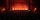A concert will take place at the stadium. If they sesolddll 1/4 ticket on the first day, 2/5 of the rest on the second day, and 17,100 tickets left in the box office. What is the capacity of the stadium?
• The temperature 14The temperature is 12°C lower than 4 hours ago. a. What is the average temperature change per hour? b. Write an integer equation for the situation.
• Washing machine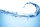How much did the washing machine cost, did we pay an advance payment for it in the amount of 1/5 of the total price of the washing machine and then installments in the total amount of 320 €?
• AlexandraAlexandra made a rectangular quilt the measured 3 1/4. 2 3/4 feet in width. What is the area of the quilt in square feet? Write an equation to solve.
• Three integersThree consecutive integers add up to 51. what are these integers?
• Original numberJoe divided a number by 2 1/4 to get 2/3. What was the original number?
• The product 2The product of two functions is 10. If one of them is 2 1/3, find the other one.
• Fraction equationEx over four is equal to two and one-fifth times ex plus three over ten. What is ex>
• Frac equation v23.80 is two fifths of a quarter sum of money. How much money is there?
• Two fraction equation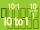Find d value of q in the equation 30/16=q/48.
• A class IV.CIn a class 2/5 were boys. 30 were girls. How many more girls than boys are there?
• Six times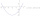6 times a number has 2 subtracted from it. The result it thrilled . the answer is the same if you add 6 to twice the number and multiply the result by 2
• Over fractionSolve equation: p over 5 equals fraction numerator 7 p over denominator 6 end fraction plus 5
• Taken away from number18 is taken away from 8 times of a number is 30. Find the number.
• The sum 14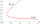The sum of five times a number and 10 is equal to the difference of the number and 10.
• SixteenSixteen minus the quotient of 12 and what number is thirteen?
• Fraction eq2/3x + 5/7 = 1/2x + 22/21
• Two numbers 11The sum of two rational numbers is (-2). If one of them is 3/5, find the other.

Do you have an exciting math question or word problem that you can't solve? Ask a question or post a math problem, and we can try to solve it.

We will send a solution to your e-mail address. Solved examples are also published here. Please enter the e-mail correctly and check whether you don't have a full mailbox.

Do you have a linear equation or system of equations and looking for its solution? Or do you have a quadratic equation? Equations Math problems. Examples for 7th grade (seventh).